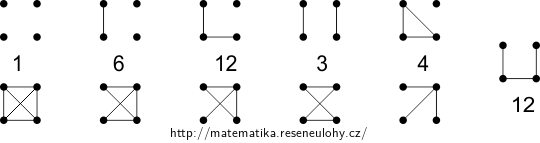## Graphs with four vertices

Find all non-isomorphic graphs with four vertices.

For each of these, determine how many isomorphic counterparts it would have if the set of vertices were fixed (e.g. $$\{u,v,w,x\}$$).

• #### Solution

The graphs are in the following picture. Graphs above each other are mutually complementary; only the last group $$P_4$$ is its own complement.

Complementary graphs have isomorphism classes of the same size, which is given below.Concretely:
• There is one empty graph (every permutation of vertices gives the same graph).
• There are six graphs with a single edge – we can choose a pair (i.e. an edge) from a four-element set in $$\binom{4}{2}=6$$ ways.
• There are twelve graphs isomorphic to $$P_3\cup K_1$$ – there are four choices for the isolated vertex, and then three choices from the remaining vertices for the midpoint of $$P_3$$.
• There are only three matchings: there are three choices for the the neighbor of $$u$$.
• There are four graphs $$K_{1{,}3}$$ – the choice is for the central vertex.
• There are $$\frac{4!}{2}$$ paths – each permutation gives an arrangement of vertices into a sequence, and two sequences yield the same graph if they are mutually symmetric.